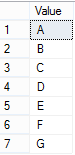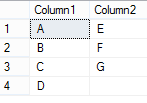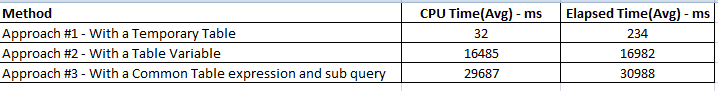# Divide rows of a column in two columns 5

In this post “Divide rows in two columns”, we are going to learn a trick to divide a column’s rows in two columns. We have find the total number of rows and then distribute it in two columns, For example, a table with a column containing 6 rows, will split in two columns, each of 3 rows. In case of odd numbers of rows in the column, in second column, a blank string should appear for last entry. Lets have a look on the below table and required output to understand this;

```SELECT * FROM dbo.DemoTable
```Required outputYou can see in above figures, we have 7 rows in our demo table and these rows have been distributed in two columns. Hence, we have a odd count for total rows, last row in column2 has a blank value. Below is the demo table with sample data.

#### Create a demo table with sample data

```IF(OBJECT_ID('dbo.DemoTable') IS NOT NULL)
DROP TABLE dbo.DemoTable
GO
CREATE TABLE dbo.DemoTable
(
Value VARCHAR(100)
)
GO
INSERT INTO dbo.DemoTable(Value)
SELECT 'A' UNION ALL SELECT 'B' UNION ALL SELECT 'C' UNION ALL SELECT 'D' UNION ALL SELECT 'E' UNION ALL SELECT 'F' UNION ALL SELECT 'G'
GO
```

In this demo, we are going to use SerialNumber table as we do in our most of the articles. Below is the structure of the SerialNumber table, with 100,000 rows.

#### SerailNumber table with 100,000 rows

```CREATE TABLE dbo.SerialNumber
(
SNo INT NOT NULL PRIMARY KEY
)
GO
INSERT INTO dbo.SerialNumber
(SNo)
SELECT TOP 100000 ROW_NUMBER() OVER(ORDER BY (SELECT 0)) FROM SYS.COLUMNS A CROSS JOIN SYS.COLUMNS B
SELECT * FROM SerialNumber
```

## Splitting rows in two columns

We can achieve this task in many ways and here we are going to discuss some of them. We will also compare the performance at the end of this post.

### Approach #1 – With a Temporary Table

```--Create a temp table
IF(OBJECT_ID('tempdb..#tbl') IS NOT NULL)
DROP TABLE #TBL
GO
CREATE TABLE #Tbl
(
SEQ INT PRIMARY KEY,
Value VARCHAR(100)
)
GO
--Generate row number for all the records of DemoTable and insert this in temp table
INSERT INTO #Tbl(SEQ, Value)
SELECT ROW_NUMBER() OVER(ORDER BY (SELECT 0)) AS SEQ, Value FROM dbo.DemoTable
GO
--Script to split rows with the help of a counter variable
DECLARE @Cnt INT = 1
SELECT @Cnt = CEILING(COUNT(1) / 2.0) FROM dbo.DemoTable

SELECT
ISNULL(DT1.Value, '') AS Column1, ISNULL(DT2.Value, '') AS Column2
FROM SerialNumber SN
LEFT JOIN #tbl DT1 ON DT1.SEQ = SN.SNo AND DT1.SEQ <= @Cnt
LEFT JOIN #tbl DT2 ON DT2.SEQ = SN.SNo + @Cnt AND DT2.SEQ > @Cnt
WHERE SNO <= @Cnt
```

### Approach #2 – With a Table Variable

```DECLARE @Tbl TABLE
(
SEQ INT PRIMARY KEY,
Value VARCHAR(100)
)
INSERT INTO @Tbl(SEQ, Value)
SELECT ROW_NUMBER() OVER(ORDER BY (SELECT 0)) AS SEQ, Value FROM dbo.DemoTable

DECLARE @Cnt INT = 1
SELECT @Cnt = CEILING(COUNT(1) / 2.0) FROM dbo.DemoTable

SELECT
SNo
, ISNULL(DT1.Value, '') AS Column1, ISNULL(DT2.Value, '') AS Column2
FROM SerialNumber SN
LEFT JOIN @tbl DT1 ON DT1.SEQ = SN.SNo AND DT1.SEQ <= @Cnt
LEFT JOIN @tbl DT2 ON DT2.SEQ = SN.SNo + @Cnt AND DT2.SEQ > @Cnt
WHERE SNO <= @Cnt
```

### Approach #3 – With a Common Table expression and sub query

```DECLARE @Cnt INT = 1
SELECT @Cnt = CEILING(COUNT(1) / 2.0) FROM dbo.DemoTable
;WITH CTE AS
(
SELECT ROW_NUMBER() OVER(ORDER BY (SELECT 0)) AS SEQ, Value FROM dbo.DemoTable
)
SELECT
SNo, ISNULL((SELECT Value FROM CTE DT1 WHERE DT1.SEQ = SN.SNo AND DT1.SEQ <= @Cnt), '') AS Column1,
ISNULL((SELECT Value FROM CTE DT2 WHERE DT2.SEQ = SN.SNo + @Cnt AND DT2.SEQ > @Cnt), '') AS Column2
FROM SerialNumber SN
WHERE SNO <= @Cnt
```

### Performance comparison chart

Below is the performance comparison chart for all three approaches with 10,000 sample rows in Demo Table. In this chart, you can see that the Temp Table approach is the fastest one.## Conclusion

In above logic, we have used SerialNumber table to get half of the rows in column1 and other half in column2. We have also used CEILING function to handle the odd and even number of rows in same way.

Rate This
[Total: 3   Average: 4/5]Gopal is a passionate Data Engineer and Data Analyst. He has implemented many end to end solutions using Big Data, Machine Learning, OLAP, OLTP, and cloud technologies. He loves to share his experience at https://www.sqlrelease.com/. Connect with Gopal on LinkedIn at https://www.linkedin.com/in/ergkranjan/.

This site uses Akismet to reduce spam. Learn how your comment data is processed.

## 5 thoughts on “Divide rows of a column in two columns”

•Gerald Britton

Simpler, using CTE:

<code.
DECLARE @Cnt INT = 1

SELECT @Cnt = CEILING(COUNT(1) / 2.0)
FROM dbo.SerialNumber;

WITH CTE
AS (
SELECT SNo
FROM dbo.SerialNumber
)
SELECT c1.SNo c1seq
, c2.SNo c2seq
FROM CTE c1
LEFT JOIN cte c2 ON c2.SNo = c1.SNo + @cnt
WHERE c1.SNo <= @cnt
ORDER BY c1seq

(50000 row(s) affected)

SQL Server Execution Times:
CPU time = 94 ms, elapsed time = 361 ms.

•Gopal Krishna Ranjan

Hi @Cathan, Thanks for raising the “Why”, which i missed completely in this post.
Once, in my short career, i was working on Fish-Bone report, where this scenario occurred and it was required to put the extracted output in two columns with equal number of rows.

•Cathan P. Kirkwood

Hello,

Great article on the how’s for this, but it’s severely lacking in the why. The data added to the rows should either be related in the same column by row, or be using the columns to reduce rows returned, though the data would be the same and wouldn’t reduce the network io.

For instance, if we were to have a record set which had invoices and the related payments as rows unioned together, and ordered by the order number then the type (invoice or payment), then adding the second records columns into the same row would prove quite useful especially if there were computed columns as well, but I’m also not sure that a CTE joined to itself where the ordernumber was the same and the type was greater wouldn’t be more efficient. And I don’t mean to criticize, but provide some insight into the uses as well as the how’s and I believe your article would be much more useful to a wider range of dba’s.

•Evert-Jan Modderkolk

What, when using this query in the 1st example?

SELECT
ISNULL(DT1.Value, ”) AS Column1, ISNULL(DT2.Value, ”) AS Column2
FROM #tbl DT1
LEFT JOIN #tbl DT2 ON DT2.SEQ = DT1.SEQ + @Cnt AND DT2.SEQ > @Cnt
WHERE DT1.SEQ <= @Cnt

•Gopal Krishna Ranjan

Hi Evert,